# Expansion Measurement of the Edge Velocity of the Bubble Nebula (NGC 7635)

0
4005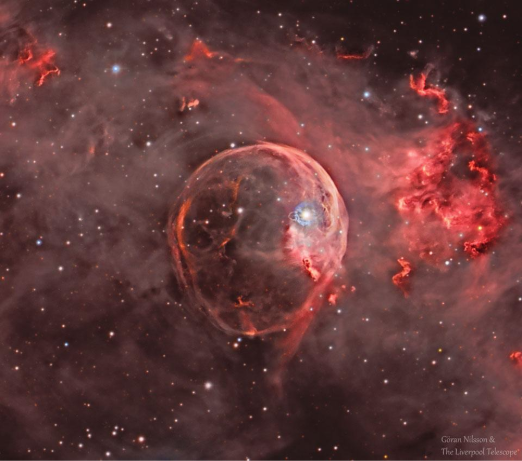## Expansion Measurement of the Edge Velocity of the Bubble Nebula (NGC 7635)

### Authors:

Clyde Xu: The Hill School, Pottstown, PA; Fritz Ruppert: Brevard High School, Breard, NC; Juan Hernandez: Alamo Heights High School, San Antonio, TX

### Abstract:

In comparison to other nebulae, the Bubble Nebula certainly has not received much attention. Not enough detailed research has been conducted on the nebula and therefore more data are needed to have a comprehensive understanding of the nebula.

According to the Hubble Telescope data from NASA in 2016, the average expansion rate of the Bubble Nebula is approximately 27,716.48 meters per second1. This is the only numerical data on its movements that the research team was able to find online. However, this simple number is not enough to describe all of its motion and activities. Due to its expansion, its velocity and expansion rates are far more complex than other nebulae. Therefore, the research team decided to conduct a more thorough research in search of more data. The purposes of this research are not only to verify NASA’s measurement, but also to discover the more information, including its overall speed and more specific expansion rates, of the nebula. In the end, the team was able to calculate the speeds and kinetic energy of the nebula, along with a new set of numbers for expansion rate. However, although the estimated expansion rates are different from that of NASA, it was concluded that both the calculations are correct.

### Introduction:

Nebulae are typically either supernova remnants or stellar nurseries. They found near the explosion of a dying star or at regions where new stars are forming2. They are absolutely beautiful and distinct in the colorful universe. The Bubble Nebula is even unique among other nebulae. It is part of the Cassiopeia constellation, approximately 11,000 light years away. It is also referred to as NGC 7635. It is located at coordinates 23h 20m 48.3s by +61° 12′ 06″, using the right ascension and declination system. It has a radius of 3.5 light years and an angular size of 15 by 8 arcminutes. However, the more substantial reason the Bubble nebula is unique is the fact that it is being powered by solar winds. The origin of the winds are the parent star of the nebula, BD+60°2522. The star continually ejects gas and therefore creates the nebula. This anomaly is what gives the nebula its distinct shape and name, and also what makes it scientifically intriguing. Its parent star, BD+60°2522, is an O-class star with an approximated surface temperature of 37 500 K3. Currently, it is estimated to be 15 times the Sun’s radius and 44 times its mass. It has an apparent magnitude of 8.7((Constellation Guide. Admin. September 04, 2014)).

### Observations and Calculations

The research team was resided at Pisgah Astronomical Research Institution (PARI) in North Carolina to gather more data. Using PARI’s 12-meter radio telescope, the following spectrum of the Bubble Nebula is obtained with an Intermediate Frequency (IF) gain of 17. The IF gain controls the IF amplifier that process the output from the nebula ((How does a radio telescope work? Radio Astronomy)).

Figure 1: Spectrum Scan of Bubble nebula produced by 12 meter radio telescope

(Please note that multiple scans are taken to ensure its accuracy. The scan above is just one of the samples.)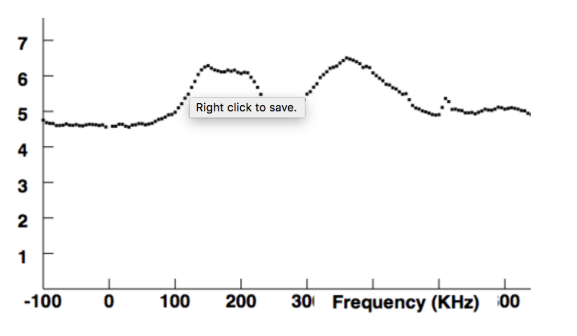In this diagram, two major curves are presented relative to the frequency on the X-axis. This is due to the expansion of the nebula. As the nebula is expanding in all directions, each curve represents a different part of the nebula. The details of this concept will be later explained in the Conclusion section. Using the Doppler effect formula,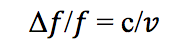the speed of the nebula could be calculated4. According to the relative minimum points of the two major curves, three different speeds are present at three different frequencies: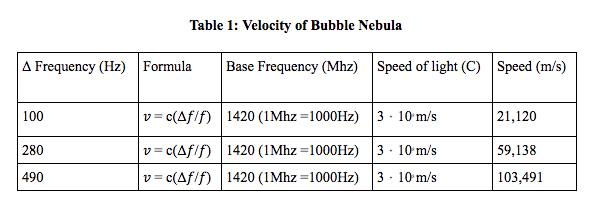The three speeds at the left columns are very inconsistent, with the largest number, 103,491, almost 5 times as big as the smallest one. So what have caused the difference in speed? The answer is expansion. As the Bubble nebula is moving, it is also expanding, causing a change in its velocity. In other words, the rate of expansion is the change in velocity, mathematically expressed mathematically as ??. Using the three values of speeds obtained earlier, the table below is easily constructed.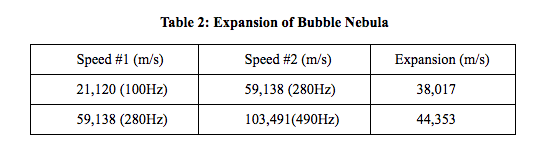With the results of the expansion calculation, the kinetic energy can also be determined. As the Sun is 99.98% of the mass of the solar system, astronomers typically use 1 Solar Mass as its mass5. Similarly, the team assumes that the mass of the nebula is roughly equal to the mass of its parent star. At its current stage, the mass of BD+60°2522 is approximate 44 Solar Masses. With this information, the kinetic energy of the nebula due to its expansion could be calculated with the formula “KE =  ½ MV2 ”. The result 7.422640 Joules, using a mass of 8.7520 x 1031 kg for the parent star and the average expansion rate of 41185 m/s.”

### Discussion and Conclusion:

The expansion rates calculated in this research are different from the data provided by the Hubble Space Telescope two years ago. Which one is wrong? The answer is neither. All measurements are found to be correct. The inconsistencies in results are mostly likely due to the chosen frequency. In this study, the team decided to use the relative minimum points rather than the midpoints of the two curves. This results in substituting different values of frequencies into the Doppler formula, and therefore different velocity and expansion rates are calculated.

The minimum frequencies of the two curves correspond to the activities at the edges of the nebula. Thus, the two expansion speeds determined in this research represent the rates at the two edges of the nebula, while the data from NASA, 27716 m/s, illustrate the expansion rate near the center of the nebula. All sets of speeds are correct – different parts of the nebula are being measured.

According to the Doppler effect, objects moving towards Earth will tend to have a lower wavelength and therefore higher frequency6. Utilizing this concept into the research, 38,017m/s is the rate of expansion on the further edge of the nebula, and 44,353 m/s is the rate at the closer edge.

Overall, the research was considered to be a success. The team was able to present more data regarding the velocity and more specific expansion rates of the nebula. A side goal of this research is actually to encourage more students conducting astronomical research in the future. Most high school students are hindered from doing astronomical research is due to the lack of access to advanced instruments. However, the completion of this study only required the 12 meter radio telescope at PARI for spectrum scans. It is evident that students would not need the most advanced telescope or satellite to conduct research in astronomy. Moreover, there are astronomical institutions that could allow students to use certain instruments. Thus, high school students should actively seek for research opportunities in astronomy.

### Acknowledgements:

The research team would like to acknowledge the support of these individuals and institutions:

The PARI Observatory

Duke University Talent Identification Program

All instructors

Specifically:

Dr. Michael Castelaz for physics and mathematics assistance

Timothy DeLisle for technical support

Christi Whitworth for general guidance

### Reference:

Morrow, A. (2016, April 21). Hubble Sees a Star ‘Inflating’ a Giant Bubble. Retrieved from https://www.nasa.gov/feature/goddard/2016/hubble-sees-a-star-inflating-a-giant-bubble

Newman, P. (2018, Feb. 5). Astronomy Picture of the Day. Retrieved from https://apod.nasa.gov/apod/ap180205.html

Malik, T. (2016, April 21). A ‘Bubble’ for Hubble: Iconic Telescope Snaps Spectacular

Nilsson, G. (2018, Feb. 5). NGC 7635: The Bubble Nebula Expanding. Retrieved from

https://science.nasa.gov/ngc-7635-bubble-nebula-expanding

https://spaceplace.nasa.gov/nebula/en/